# Chapter 10: Differential Calculus Differentiability and Methods of Differentiation - Online Test

Q1. d/ dx (2/π sin x° ) is
Answer : Option B
Explaination / Solution:Q2. If y = f (x2 + 2) and f '(3) = 5, then dy/dx at x = 1 is
Answer : Option D
Explaination / Solution: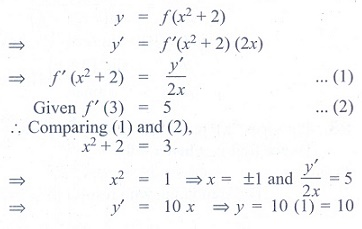Q3. If y = 1/4  u4 , u = 2/3  x3 + 5, then dy/dx is
Answer : Option C
Explaination / Solution: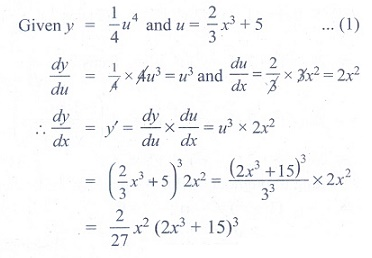Q4. If f (x) = x2 − 3x , then the points at which f (x) = f '(x) are
Answer : Option C
Explaination / Solution: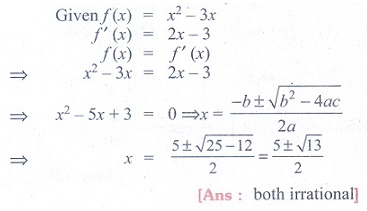Q5. If y = 1/ a − z , then dz/ dy is
Answer : Option A
Explaination / Solution:Q6. If y = cos(sin x2), then dy/dx at x = [π/2] is
Answer : Option D
Explaination / Solution:Q7. If y = mx + c and f (0) = f '(0) = 1, then f (2) is
Answer : Option C
Explaination / Solution: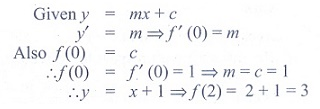Q8. If f (x) = x tan−1x , then f '(1) is
Answer : Option B
Explaination / Solution: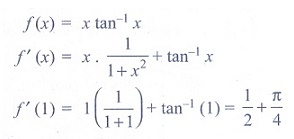Q9. d/ dx (ex +5logx) is
Answer : Option A
Explaination / Solution:Q10. If the derivative of (ax − 5)e3x at x = 0 is -13, then the value of a is
Answer : Option D
Explaination / Solution: